# 12.1 The ellipse  (Page 9/16)

 Page 9 / 16

$4{x}^{2}+16{y}^{2}=1$

$\frac{{\left(x-2\right)}^{2}}{49}+\frac{{\left(y-4\right)}^{2}}{25}=1$

$\frac{{\left(x-2\right)}^{2}}{{7}^{2}}+\frac{{\left(y-4\right)}^{2}}{{5}^{2}}=1;\text{\hspace{0.17em}}$ Endpoints of major axis $\text{\hspace{0.17em}}\left(9,4\right),\left(-5,4\right).\text{\hspace{0.17em}}$ Endpoints of minor axis $\text{\hspace{0.17em}}\left(2,9\right),\left(2,-1\right).\text{\hspace{0.17em}}$ Foci at $\text{\hspace{0.17em}}\left(2+2\sqrt{6},4\right),\left(2-2\sqrt{6},4\right).$

$\frac{{\left(x-2\right)}^{2}}{81}+\frac{{\left(y+1\right)}^{2}}{16}=1$

$\frac{{\left(x+5\right)}^{2}}{4}+\frac{{\left(y-7\right)}^{2}}{9}=1$

$\frac{{\left(x+5\right)}^{2}}{{2}^{2}}+\frac{{\left(y-7\right)}^{2}}{{3}^{2}}=1;\text{\hspace{0.17em}}$ Endpoints of major axis $\text{\hspace{0.17em}}\left(-5,10\right),\left(-5,4\right).\text{\hspace{0.17em}}$ Endpoints of minor axis $\text{\hspace{0.17em}}\left(-3,7\right),\left(-7,7\right).\text{\hspace{0.17em}}$ Foci at $\text{\hspace{0.17em}}\left(-5,7+\sqrt{5}\right),\left(-5,7-\sqrt{5}\right).$

$\frac{{\left(x-7\right)}^{2}}{49}+\frac{{\left(y-7\right)}^{2}}{49}=1$

$4{x}^{2}-8x+9{y}^{2}-72y+112=0$

$\frac{{\left(x-1\right)}^{2}}{{3}^{2}}+\frac{{\left(y-4\right)}^{2}}{{2}^{2}}=1;\text{\hspace{0.17em}}$ Endpoints of major axis $\text{\hspace{0.17em}}\left(4,4\right),\left(-2,4\right).\text{\hspace{0.17em}}$ Endpoints of minor axis $\text{\hspace{0.17em}}\left(1,6\right),\left(1,2\right).\text{\hspace{0.17em}}$ Foci at $\text{\hspace{0.17em}}\left(1+\sqrt{5},4\right),\left(1-\sqrt{5},4\right).$

$9{x}^{2}-54x+9{y}^{2}-54y+81=0$

$4{x}^{2}-24x+36{y}^{2}-360y+864=0$

$\frac{{\left(x-3\right)}^{2}}{{\left(3\sqrt{2}\right)}^{2}}+\frac{{\left(y-5\right)}^{2}}{{\left(\sqrt{2}\right)}^{2}}=1;\text{\hspace{0.17em}}$ Endpoints of major axis $\text{\hspace{0.17em}}\left(3+3\sqrt{2},5\right),\left(3-3\sqrt{2},5\right).\text{\hspace{0.17em}}$ Endpoints of minor axis $\text{\hspace{0.17em}}\left(3,5+\sqrt{2}\right),\left(3,5-\sqrt{2}\right).\text{\hspace{0.17em}}$ Foci at $\text{\hspace{0.17em}}\left(7,5\right),\left(-1,5\right).$

$4{x}^{2}+24x+16{y}^{2}-128y+228=0$

$4{x}^{2}+40x+25{y}^{2}-100y+100=0$

$\frac{{\left(x+5\right)}^{2}}{{\left(5\right)}^{2}}+\frac{{\left(y-2\right)}^{2}}{{\left(2\right)}^{2}}=1;\text{\hspace{0.17em}}$ Endpoints of major axis $\text{\hspace{0.17em}}\left(0,2\right),\left(-10,2\right).\text{\hspace{0.17em}}$ Endpoints of minor axis $\text{\hspace{0.17em}}\left(-5,4\right),\left(-5,0\right).\text{\hspace{0.17em}}$ Foci at $\text{\hspace{0.17em}}\left(-5+\sqrt{21},2\right),\left(-5-\sqrt{21},2\right).$

${x}^{2}+2x+100{y}^{2}-1000y+2401=0$

$4{x}^{2}+24x+25{y}^{2}+200y+336=0$

$\frac{{\left(x+3\right)}^{2}}{{\left(5\right)}^{2}}+\frac{{\left(y+4\right)}^{2}}{{\left(2\right)}^{2}}=1;\text{\hspace{0.17em}}$ Endpoints of major axis $\text{\hspace{0.17em}}\left(2,-4\right),\left(-8,-4\right).\text{\hspace{0.17em}}$ Endpoints of minor axis $\text{\hspace{0.17em}}\left(-3,-2\right),\left(-3,-6\right).\text{\hspace{0.17em}}$ Foci at $\text{\hspace{0.17em}}\left(-3+\sqrt{21},-4\right),\left(-3-\sqrt{21},-4\right).$

$9{x}^{2}+72x+16{y}^{2}+16y+4=0$

For the following exercises, find the foci for the given ellipses.

$\frac{{\left(x+3\right)}^{2}}{25}+\frac{{\left(y+1\right)}^{2}}{36}=1$

Foci $\text{\hspace{0.17em}}\left(-3,-1+\sqrt{11}\right),\left(-3,-1-\sqrt{11}\right)$

$\frac{{\left(x+1\right)}^{2}}{100}+\frac{{\left(y-2\right)}^{2}}{4}=1$

${x}^{2}+{y}^{2}=1$

Focus $\text{\hspace{0.17em}}\left(0,0\right)$

${x}^{2}+4{y}^{2}+4x+8y=1$

$10{x}^{2}+{y}^{2}+200x=0$

Foci $\text{\hspace{0.17em}}\left(-10,30\right),\left(-10,-30\right)$

## Graphical

For the following exercises, graph the given ellipses, noting center, vertices, and foci.

$\frac{{x}^{2}}{25}+\frac{{y}^{2}}{36}=1$

$\frac{{x}^{2}}{16}+\frac{{y}^{2}}{9}=1$

Center $\text{\hspace{0.17em}}\left(0,0\right),\text{\hspace{0.17em}}$ Vertices $\text{\hspace{0.17em}}\left(4,0\right),\left(-4,0\right),\left(0,3\right),\left(0,-3\right),\text{\hspace{0.17em}}$ Foci $\text{\hspace{0.17em}}\left(\sqrt{7},0\right),\left(-\sqrt{7},0\right)$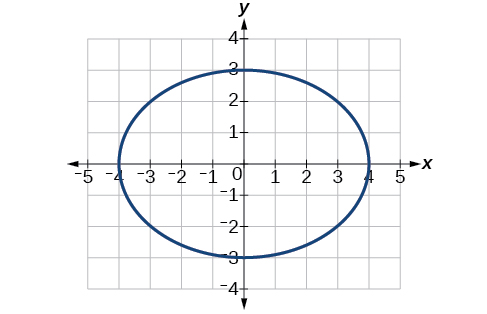$4{x}^{2}+9{y}^{2}=1$

$81{x}^{2}+49{y}^{2}=1$

Center $\text{\hspace{0.17em}}\left(0,0\right),\text{\hspace{0.17em}}$ Vertices $\text{\hspace{0.17em}}\left(\frac{1}{9},0\right),\left(-\frac{1}{9},0\right),\left(0,\frac{1}{7}\right),\left(0,-\frac{1}{7}\right),\text{\hspace{0.17em}}$ Foci $\text{\hspace{0.17em}}\left(0,\frac{4\sqrt{2}}{63}\right),\left(0,-\frac{4\sqrt{2}}{63}\right)$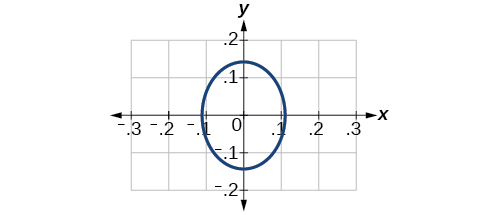$\frac{{\left(x-2\right)}^{2}}{64}+\frac{{\left(y-4\right)}^{2}}{16}=1$

$\frac{{\left(x+3\right)}^{2}}{9}+\frac{{\left(y-3\right)}^{2}}{9}=1$

Center $\text{\hspace{0.17em}}\left(-3,3\right),\text{\hspace{0.17em}}$ Vertices $\text{\hspace{0.17em}}\left(0,3\right),\left(-6,3\right),\left(-3,0\right),\left(-3,6\right),\text{\hspace{0.17em}}$ Focus $\text{\hspace{0.17em}}\left(-3,3\right)\text{\hspace{0.17em}}$

Note that this ellipse is a circle. The circle has only one focus, which coincides with the center.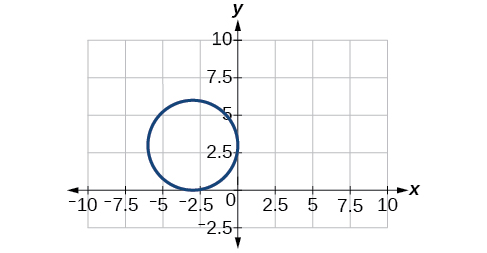$\frac{{x}^{2}}{2}+\frac{{\left(y+1\right)}^{2}}{5}=1$

$4{x}^{2}-8x+16{y}^{2}-32y-44=0$

Center $\text{\hspace{0.17em}}\left(1,1\right),\text{\hspace{0.17em}}$ Vertices $\text{\hspace{0.17em}}\left(5,1\right),\left(-3,1\right),\left(1,3\right),\left(1,-1\right),\text{\hspace{0.17em}}$ Foci $\text{\hspace{0.17em}}\left(1,1+4\sqrt{3}\right),\left(1,1-4\sqrt{3}\right)$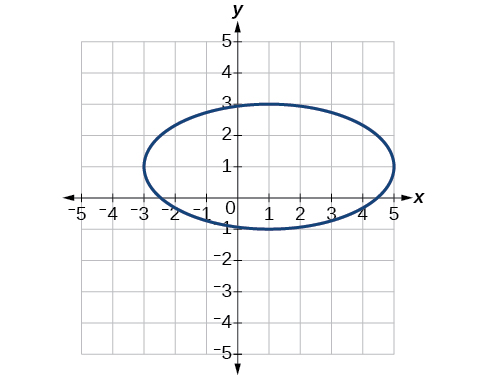${x}^{2}-8x+25{y}^{2}-100y+91=0$

${x}^{2}+8x+4{y}^{2}-40y+112=0$

Center $\text{\hspace{0.17em}}\left(-4,5\right),\text{\hspace{0.17em}}$ Vertices $\text{\hspace{0.17em}}\left(-2,5\right),\left(-6,4\right),\left(-4,6\right),\left(-4,4\right),\text{\hspace{0.17em}}$ Foci $\text{\hspace{0.17em}}\left(-4+\sqrt{3},5\right),\left(-4-\sqrt{3},5\right)$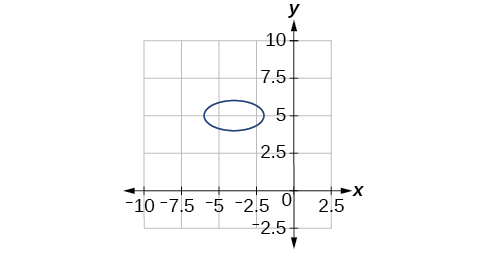$64{x}^{2}+128x+9{y}^{2}-72y-368=0$

$16{x}^{2}+64x+4{y}^{2}-8y+4=0$

Center $\text{\hspace{0.17em}}\left(-2,1\right),\text{\hspace{0.17em}}$ Vertices $\text{\hspace{0.17em}}\left(0,1\right),\left(-4,1\right),\left(-2,5\right),\left(-2,-3\right),\text{\hspace{0.17em}}$ Foci $\text{\hspace{0.17em}}\left(-2,1+2\sqrt{3}\right),\left(-2,1-2\sqrt{3}\right)$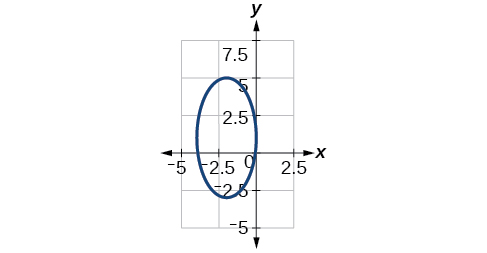$100{x}^{2}+1000x+{y}^{2}-10y+2425=0$

$4{x}^{2}+16x+4{y}^{2}+16y+16=0$

Center $\text{\hspace{0.17em}}\left(-2,-2\right),\text{\hspace{0.17em}}$ Vertices $\text{\hspace{0.17em}}\left(0,-2\right),\left(-4,-2\right),\left(-2,0\right),\left(-2,-4\right),\text{\hspace{0.17em}}$ Focus $\text{\hspace{0.17em}}\left(-2,-2\right)$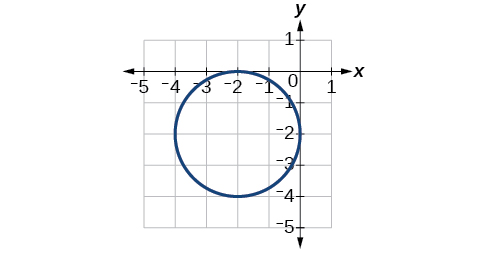For the following exercises, use the given information about the graph of each ellipse to determine its equation.

Center at the origin, symmetric with respect to the x - and y -axes, focus at $\text{\hspace{0.17em}}\left(4,0\right),$ and point on graph $\text{\hspace{0.17em}}\left(0,3\right).$

Center at the origin, symmetric with respect to the x - and y -axes, focus at $\text{\hspace{0.17em}}\left(0,-2\right),$ and point on graph $\text{\hspace{0.17em}}\left(5,0\right).$

$\frac{{x}^{2}}{25}+\frac{{y}^{2}}{29}=1$

Center at the origin, symmetric with respect to the x - and y -axes, focus at $\text{\hspace{0.17em}}\left(3,0\right),$ and major axis is twice as long as minor axis.

Center $\text{\hspace{0.17em}}\left(4,2\right)$ ; vertex $\text{\hspace{0.17em}}\left(9,2\right)$ ; one focus: $\text{\hspace{0.17em}}\left(4+2\sqrt{6},2\right)$ .

$\frac{{\left(x-4\right)}^{2}}{25}+\frac{{\left(y-2\right)}^{2}}{1}=1$

Center $\text{\hspace{0.17em}}\left(3,5\right)$ ; vertex $\text{\hspace{0.17em}}\left(3,11\right)$ ; one focus:

Center $\text{\hspace{0.17em}}\left(-3,4\right)$ ; vertex $\text{\hspace{0.17em}}\left(1,4\right)$ ; one focus: $\text{\hspace{0.17em}}\left(-3+2\sqrt{3},4\right)$

$\frac{{\left(x+3\right)}^{2}}{16}+\frac{{\left(y-4\right)}^{2}}{4}=1$

For the following exercises, given the graph of the ellipse, determine its equation.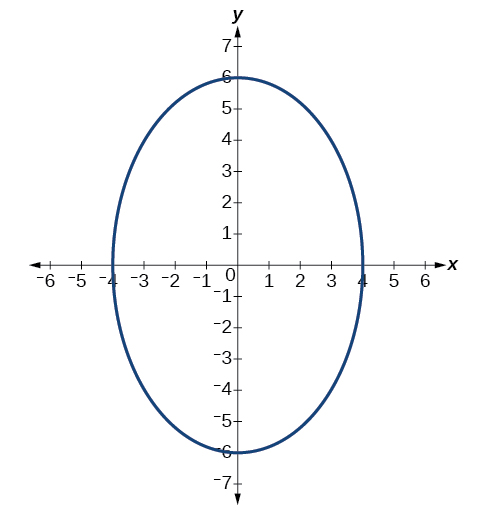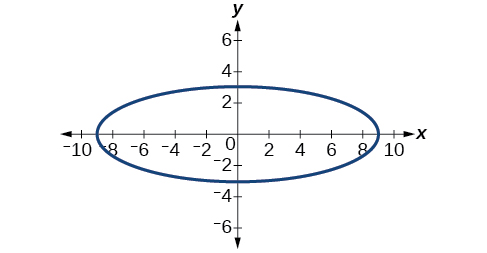$\frac{{x}^{2}}{81}+\frac{{y}^{2}}{9}=1$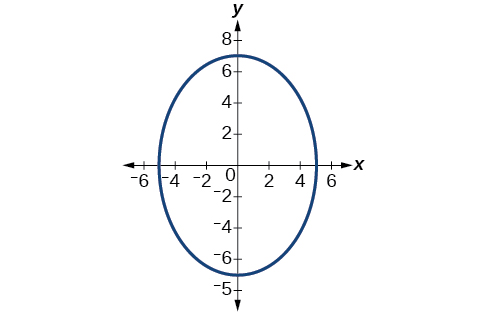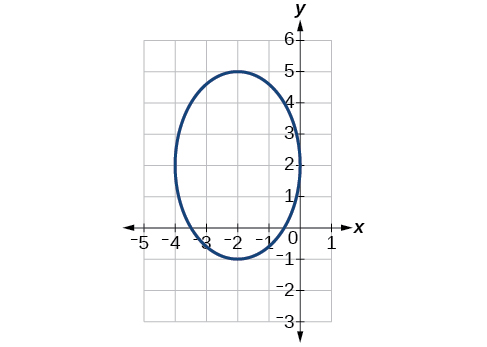$\frac{{\left(x+2\right)}^{2}}{4}+\frac{{\left(y-2\right)}^{2}}{9}=1$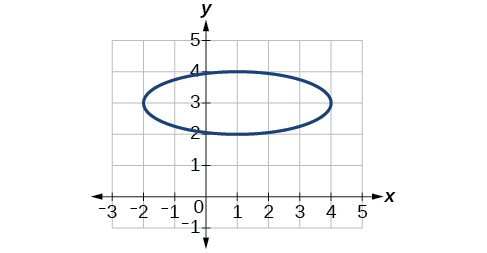## Extensions

For the following exercises, find the area of the ellipse. The area of an ellipse is given by the formula $\text{\hspace{0.17em}}\text{Area}=a\cdot b\cdot \pi .$

$\frac{{\left(x-3\right)}^{2}}{9}+\frac{{\left(y-3\right)}^{2}}{16}=1$

$\frac{{\left(x+6\right)}^{2}}{16}+\frac{{\left(y-6\right)}^{2}}{36}=1$

$\frac{{\left(x+1\right)}^{2}}{4}+\frac{{\left(y-2\right)}^{2}}{5}=1$

$4{x}^{2}-8x+9{y}^{2}-72y+112=0$

$9{x}^{2}-54x+9{y}^{2}-54y+81=0$

## Real-world applications

Find the equation of the ellipse that will just fit inside a box that is 8 units wide and 4 units high.

Find the equation of the ellipse that will just fit inside a box that is four times as wide as it is high. Express in terms of $\text{\hspace{0.17em}}h,$ the height.

$\frac{{x}^{2}}{4{h}^{2}}+\frac{{y}^{2}}{\frac{1}{4}{h}^{2}}=1$

An arch has the shape of a semi-ellipse (the top half of an ellipse). The arch has a height of 8 feet and a span of 20 feet. Find an equation for the ellipse, and use that to find the height to the nearest 0.01 foot of the arch at a distance of 4 feet from the center.

An arch has the shape of a semi-ellipse. The arch has a height of 12 feet and a span of 40 feet. Find an equation for the ellipse, and use that to find the distance from the center to a point at which the height is 6 feet. Round to the nearest hundredth.

$\frac{{x}^{2}}{400}+\frac{{y}^{2}}{144}=1$ . Distance = 17.32 feet

A bridge is to be built in the shape of a semi-elliptical arch and is to have a span of 120 feet. The height of the arch at a distance of 40 feet from the center is to be 8 feet. Find the height of the arch at its center.

A person in a whispering gallery standing at one focus of the ellipse can whisper and be heard by a person standing at the other focus because all the sound waves that reach the ceiling are reflected to the other person. If a whispering gallery has a length of 120 feet, and the foci are located 30 feet from the center, find the height of the ceiling at the center.

Approximately 51.96 feet

A person is standing 8 feet from the nearest wall in a whispering gallery. If that person is at one focus, and the other focus is 80 feet away, what is the length and height at the center of the gallery?

use the properties of logarithms to rewrite as a single logarithmic expression 2+log base 5 t divided by log base 5 17
(cos²x +tan²x)÷(sec²x+cos²x)
Solve the problem 3x^2_2x+4=0
can anyone recommend an app or. website to help me refresh my knowledge and abilities? I stopped learning more about calc and trig in the 90's
if 6x=-2 find value of 6/x
l don't know
Aman
I don't know
Aman
l don't know
Aman
I don't know
Aman
x=.333333333334, so 6/x= 18±
scott
x=.33333333334± 6/x=18±
scott
that is strange, I don't remember inserting the À. the result I got was x= 3333333334± 6/x=18±
scott
I need glasses
scott
X=(-1÷3) X equals minus one third
Melvin
how can I get the answer if don't know it
x=-3
Dani
-18
Nwaneri
if sin15°=√p, express the following in terms of p
I am learning and does someone have the time to tell me what or where this particular equation would be used?
scott
prove sin²x+cos²x=3+cos4x
the difference between two signed numbers is -8.if the minued is 5,what is the subtrahend
the difference between two signed numbers is -8.if the minuend is 5.what is the subtrahend
jeramie
what are odd numbers
numbers that leave a remainder when divided by 2
Thorben
1,3,5,7,... 99,...867
Thorben
7%2=1, 679%2=1, 866245%2=1
Thorben
the third and the seventh terms of a G.P are 81 and 16, find the first and fifth terms.
if a=3, b =4 and c=5 find the six trigonometric value sin
Ans
pls how do I factorize x⁴+x³-7x²-x+6=0
in a function the input value is called
how do I test for values on the number line
if a=4 b=4 then a+b=
a+b+2ab
Kin
commulative principle
a+b= 4+4=8
Mimi
If a=4 and b=4 then we add the value of a and b i.e a+b=4+4=8.
Tariq

#### Get Jobilize Job Search Mobile App in your pocket Now!ByByBy Heather McAvoyBy Madison ChristianBy Vanessa SoledadBy Mackenzie WilcoxByBy OpenStaxBy Candice ButtsBy OpenStaxByBy OpenStax